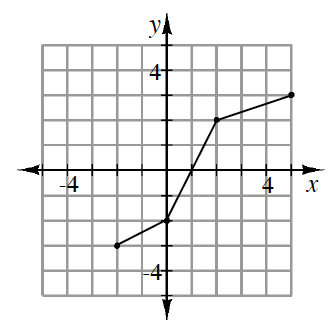### Home > CCA2 > Chapter 5 > Lesson 5.1.2 > Problem5-33

5-33.

The function $f(x)$ is represented in the graph below. Draw a graph of its inverse function. Be sure to state the domain and range for both $f(x)$ and $f^{−1}(x)$.Draw the line $y=x$ and then reflect $f(x)$ across that line, or reverse the coordinates of the points.

For $f(x)$ the domain is: $−2\le x\le5$, and the range is: $−3\le y\le3$.

Use the eTool below to graph the inverse function.
Click the link at right for the full version of the eTool: 5-33 HW eTool